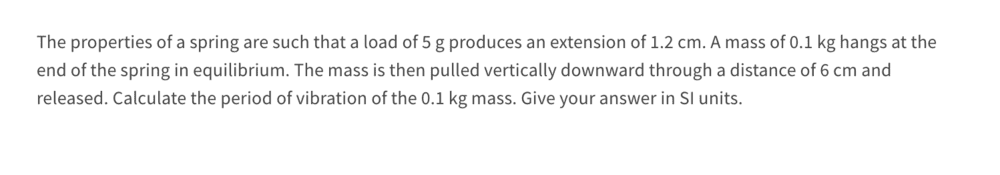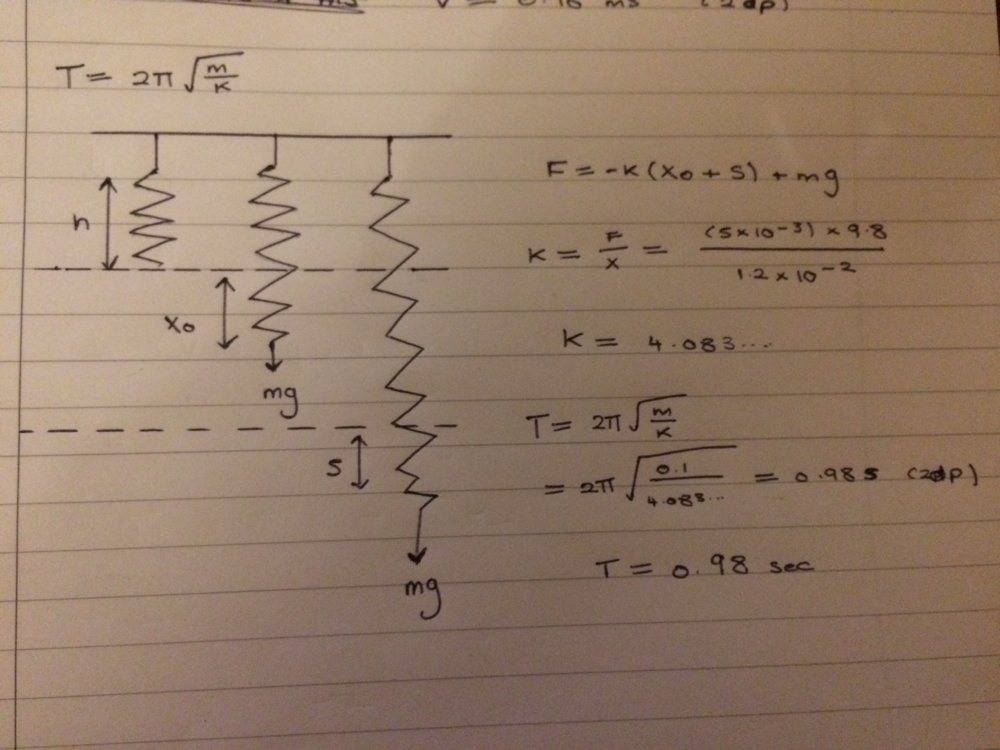# Time period of a mass spring system

Bolter
Homework Statement:
Calculate the time period of mass spring system
Relevant Equations:
See belowI have attempted to draw a sketch of this but can't see how the data they gave me help to find time period

This is what value I have ended up getting but I believe is wrongMuch appreciated for any help!

Homework Helper
Gold Member
The period looks OK to me.

Bolter
The period looks OK to me.

Thanks but what threw me off here was the extra info that was provided in the question such as the mass pulling down the spring by a distance of 6cm. I thought I had to use this 6cm somewhere in my calculation?

Homework Helper
This is a feature of the simple harmonic motion (which is the one that spring has) that is that the period (time between oscillations) is independent on the amplitude (how big the oscillations are) this feature is not true in general, for example, is not true for a pendulum (although is a good approximation for small-angle oscillations)